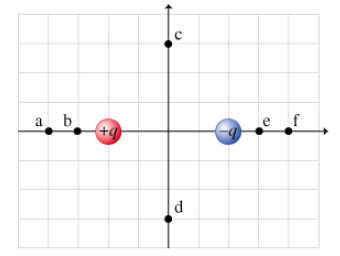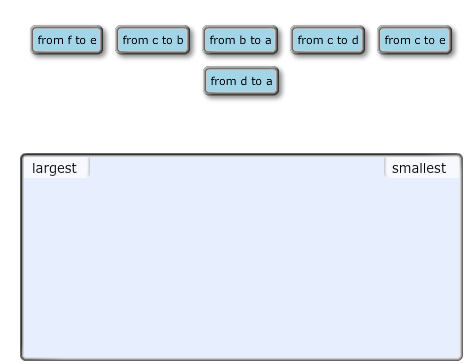# Problem: In the diagram below, there are two charges of +q and - q and six points (a through f) at various distances from the two charges (figure 1) You will be asked to rank changes in the electric potential along paths between pairs of points. Using the diagram, rank each of the given paths on the basis of the change in electric potential. Rank the largest-magnitude positive change (increase in electric potential) as largest and the largest-magnitude negative change (decrease in electric potential) as smallest. Rank from largest to smallest. To rank items as equivalent, overlap them

###### FREE Expert Solution

The change in potential:

$\overline{){\mathbf{∆}}{\mathbf{V}}{\mathbf{=}}{{\mathbf{V}}}_{{\mathbf{f}}}{\mathbf{-}}{{\mathbf{V}}}_{{\mathbf{0}}}}$

V = kq/r

In terms of kq/r

From f to e, ΔV = - 4/5 - (-1/3) = - 7/15 = - 0.467

From c to b, ΔV = 4/5 - 0 = 0.8

91% (12 ratings)###### Problem Details

In the diagram below, there are two charges of +q and - q and six points (a through f) at various distances from the two charges (figure 1) You will be asked to rank changes in the electric potential along paths between pairs of points.Using the diagram, rank each of the given paths on the basis of the change in electric potential. Rank the largest-magnitude positive change (increase in electric potential) as largest and the largest-magnitude negative change (decrease in electric potential) as smallest. Rank from largest to smallest. To rank items as equivalent, overlap themFrequently Asked Questions

What scientific concept do you need to know in order to solve this problem?

Our tutors have indicated that to solve this problem you will need to apply the Electric Potential concept. You can view video lessons to learn Electric Potential. Or if you need more Electric Potential practice, you can also practice Electric Potential practice problems.